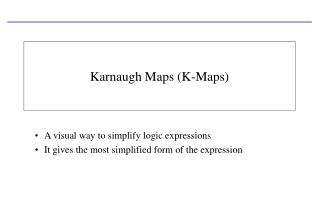DownloadDownload PresentationKarnaugh Maps (K-Maps)

# Karnaugh Maps (K-Maps)

Télécharger la présentation## Karnaugh Maps (K-Maps)

- - - - - - - - - - - - - - - - - - - - - - - - - - - E N D - - - - - - - - - - - - - - - - - - - - - - - - - - -
##### Presentation Transcript

1. Karnaugh Maps (K-Maps) A visual way to simplify logic expressions It gives the most simplified form of the expression

2. Rules to obtain the most simplified expression • Simplification of logic expression using Boolean algebra is awkward because: • it lacks specific rules to predict the most suitable next step in the simplification process • it is difficult to determine whether the simplest form has been achieved. • A Karnaugh map is a graphical method used to obtained the most simplified form of an expression in a standard form (Sum-of-Products or Product-of-Sums). • The simplest form of an expression is the one that has the minimum number of terms with the least number of literals (variables) in each term. • By simplifying an expression to the one that uses the minimum number of terms, we ensure that the function will be implemented with the minimum number of gates. • By simplifying an expression to the one that uses the least number of literals for each terms, we ensure that the function will be implemented with gates that have the minimum number of inputs.

3. Three-Variable K-Maps

4. Three-Variable K-Map Examples

5. Four-Variable K-Maps

6. Four-Variable K-Maps

7. Four-Variable K-Maps Examples

8. Four-Variable K-Maps Examples

9. Four-Variable K-Maps Examples

10. Design of combinational digital circuits • Steps to design a combinational digital circuit: • From the problem statement derive the truth table • From the truth table derive the unsimplified logic expression • Simplify the logic expression • From the simplified expression draw the logic circuit • Example: Design a 3-input (A,B,C) digital circuit that will give at its output (X) a logic 1 only if the binary number formed at the input has more ones than zeros.

11. Design of combinational digital circuits (Cont.) • Example: Design a 4-input (A,B,C,D) digital circuit that will give at its output (X) a logic 1 only if the binary number formed at the input is between 2 and 9 (including).

12. Design of combinational digital circuits (Example) • Example: Design a 4-input (A,B,C,D) digital circuit that will give at its output (X) a logic 1 only if there more ones than zeros in the binary number formed at the input.